## Pressure

Subject: Science

#### Overview

Pressure is defined as force acting per unit area. Its SI unit is Pascal. This note has brief description of pressure.

##### PressurePressure is defined as force acting per unit area. Its SI unit is Pascal or N/m2. Its formula is Pressure: $\frac{Thrust (F)}{Area (A)}$

#### Thrust:

The force acting perpendicularly on a surface is called the thrust. It is a vector quantity. The SI unit is Newton (N).

#### One Pascal pressure:

One Pascal pressure is defined as the pressure exerted on a surface of area 1m2by the thrust of 1N.

Pressure depends on following factors:

• Thrust
• area

#### Proof of P = $\frac{F}{A}$

Let 'F' be the force acting perpendicularly, 'A' be the area and 'P' be the pressure exerted.

Then,

Pressure is directly proportional to the force acting perpendicularly.

Or, P ∝F.............................(i)

Pressure is inversely proportional to the area on which force acts.

P∝$\frac{1}{A}$ .............................. (ii)

Combining equation (i) and (ii), we get

P∝$\frac{F}{A}$

or, P=K$\frac{F}{A}$ [ where K is a constant ]

If 'F' is 1N, 'A' is 1m2 and the pressure is 1N/m2, then

K=1

so, P=$\frac{F}{A}$

Atmospheric Pressure

The earth is surrounded by atmosphere which is estimated to be 9600 km i.e. about 10000 km thick. As we know air is a matter that has weight. So, atmospheric pressure is the weight of air that exerts pressure on the bodies on the earth’s surface. The value of the atmospheric pressure on sea level is one atmosphere which is considered to be standard atmospheric pressure or normal pressure. Thus, the normal pressure is the pressure exerted by 760 mm long column of mercury at the temperature of 0⁰C at the sea level. It is equivalent to 1.01 × 102 Nm-2. Some units used to express atmospheric pressure are atmosphere, torr, and bar. The interrelation between them is shown below:

1 atmosphere = 760 mm of Hg = 1.01 × 105 Pa

1 torr = 1 mm of Hg = 132.322 Pa

1 bar = 106 dynes/ cm2 = 105 Pa (Approx 750 mm of Hg) = 0.987 atm

1 millibar = 100 Pa

The value of atmospheric pressure decreases as the height from the sea level increases. It is difficult for us to survive on a very low atmospheric pressure. As the pressure of air and the blood pressure inside our body is about equal, we do not feel the normal atmospheric pressure on our body. The air pressure is maintained artificially for the passengers, convenience inside airplanes. At very high altitudes, nose, ears, and eyes may bleed due to less atmospheric pressure.

Measuring Atmospheric Pressure

We can measure the atmospheric pressure by using barometers. There are mainly two types of barometer: Mercury Barometer and Aneroid Barometer. We discuss mercury barometer in this unit.

Mercury Barometer

Mercury barometer was invented by an Italian physicist named Evangelista Torricelli. It is also known as a Torricellian barometer. The barometer consists of one long glass tube filled with mercury. The upper end of the tube is closed and has vacuum above the mercury. There is a mark of scale at the side of it.

When the atmospheric pressure increases, the mercury level rises in the tube and when the atmospheric pressure inside decreases, the mercury level falls down. This is the way in which the instrument measures the atmospheric pressure.

Some Instruments Based on Atmospheric Pressure

There are many instruments which are based on the atmospheric pressure. Some of them are: Syringe, Air Pump, Water Pump, etc. Here is the short description on the structure and working methods of these instruments.

1. Syringe:
A syringe is an instrument used in medical field to inject medicines in patients’ body. It is made up of plastic or glass. It has two parts: cylinder and piston.

The cylinder is a hollow pipe-like structure connected with a nozzle at one end and open on the other end. The nozzle is fitted with a needle and the piston is adjusted inside the cylinder. The piston can be moved inside the cylinder.

Working Method
The Syringe works by the repetition of two processes: Pulling the Piston and Pushing the Piston.

- When the piston is pulled by keeping the needle inside the liquid, the volume of the cylinder increases and pressure decreases there. In this condition, the atmospheric pressure pushes the liquid inside the cylinder.

- When the piston is pushed, the volume of liquid inside the cylinder decreases and the pressure increases. It pushes the liquid out of the syringe.

2. Air Pump
An air pump is a device used to pump air in balls, toys, tyres, stoves, etc. It can be hand-operated or machine-operated. The structure alongside shows a hand operated bicycle pump with its major two parts: Cylinder and Piston.
The cylinder is a pipe like structure connected with a nozzle fitted with the tube of a bicycle. The other end is opened through which a piston is passed inside. It contains a handle, a rod, and a washer. The washer is made soft by applying oil or grease on it and it works as the valve for the pump. The grease also reduces friction while the piston slides in the cylinder.

Working Method
The air pump works by the repetition of two processes: Pulling the Piston and Pushing the Piston.

- When the piston is pulled, the volume of part A increases and it reduces pressure in this part. The air of part B at the atmospheric pressure compresses the washer and passes into A. At this time, the valve of the bicycle is closed which blocks the air of the bicycle wheel from coming out.

- When the piston is pushed, the volume of part A decreases and it increases pressure in this part. The increased pressure spreads the washer to stop the passage of air from A to B. The part of ‘A’ enters the bicycle’s wheel by opening the valve.

3. Water Pump
A water pump is a device used to pull water from the well. It can be used to pull the underground water. It consists of four parts: cylinder, piston, pipe, and handle.

The cylinder is a hollow pipe having a spout at its one side. Its one side is open and the other end is connected with a pipe. There is a valve (valve A) at the joint of the cylinder and the pipe. The pipe is taken to the source of water. A piston is fitted inside the cylinder. The piston contains a rod, washer, and valve (valve B). The outer end of the rod is connected with a handle. The handle is a first class lever that is used to pull or push the piston.

Working Method
A water pump pulls underground water through the repetition of two processes: Downstroke and Upstroke.

Downstroke: Downstroke of the piston is the condition in which the piston is pushed down when the handle of the water pump is lifted up. In the condition, the volume of part A decreases and water pressure increases in this part. The increased pressure closes the valve ‘A’ and opens the valve ‘B’. It makes the water of part ‘A’ pass into the part ‘B’ of the cylinder.
Upstroke: When the handle of the water pump is pushed down, it lifts the piston up. It is the situation of upstroke. During upstroke, the volume of part ‘A’ increases and water pressure at that part decreases. It opens valve ‘A’ and closes valve ‘B’. Thus, the water from the source enters into part ‘A’ of the cylinder. The water of part ‘B’ is lifted up and it comes out from the spout.
##### Things to remember
• Pressure is defined as force acting per unit area. Its SI unit is Pascal or N/m2. The formula Pressure is Pressure= $\frac{Thrust (F)}{Area (A)}$
• Force is an external agent that changes or tends to change the state of a body. It is a cause of pressure,It is measured in newton, dyne, etc.
• Pressure is the thrust on unit area of surface. It is the effect of force acting on unit area, measured in N/m2.
• The pressure given by the air in the atmosphere is known as atmospheric pressure.
• The standard pressure is 1 atmosphere i.e. 760 mm of Hg.
• A barometer is used to measure atmospheric pressure.
• Some instruments based on the principle of atmospheric pressure are: Syringe, Air Pump and Water Pump.
• It includes every relationship which established among the people.
• There can be more than one community in a society. Community smaller than society.
• It is a network of social relationships which cannot see or touched.
• common interests and common objectives are not necessary for society.

A sharp knife has smaller area at its edge than a blunt knife. When a force is applied on the knife, The pressure exerted by the edge of sharp knife is more than the pressure exerted by the blunt knife during cutting. Due to this reason, cutting with a sharp knife is easier than cutting with blunt knife.

When the area is increased by keeping the magnitude of force constant, the pressure exerted is decreased because pressure is inversely proportional to the area of surface that receives the force.

When a man stands with one foot the area of surface where the force is applied is decreased and as a result the pressure is increased because keeping the force constant, the pressure is inversely proportional to the area of surface that receives the force.

1. The pressure, the force applied is directly proportional to its magnitude, that receives the area.

P α F……….. (i)

2. The force that gives pressure is inversely proportional to the area that receives the force.
P α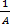………….. (ii)
From equation (i) and (ii)
P α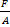P = K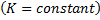If 1 N force is applied in 1 square meter area, then the pressure will be 1N/m2 and thus, k=1.

Mass of a man (m) = 60 kg

Acceleration due to gravity (g) = 10 m/s2

Weight of the man (F) = 600 N

Area occupied by one foot (A) = 150 cm2 =0.015 m2

Pressure exerted (P) =?

P ==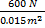=40,000 N/m2

This pressure exerted by the man on the ground when he stepped with one foot is 40,000 Pa.

Here,

Mass of Anupama (m) = 30 kg

Weight of Anupama (f) = mg = 30 kg × 10 m/s2= 300N

Surface area of a sandal (A) = 150 cm2 =0.015 m2

Pressure exerted (P) =?

P ==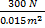=20,000 N/m2

This pressure exerted by the sandal on ground when Anupama stands on one foot is 20,000 Pa.

The pressure is defined as “the force acting perpendicularly per unit area”. The pressure is defined as the perpendicularly applied force on a unit area. The SI unit of pressure is N/m2. It is also called Pascal (pa).

Following are the examples show us the extensive use of pressure in our daily life:

1. Food is cooked faster in a pressure cooker than an open pot due to high air pressure.
2. Among the taps of same size and connected to a common pipeline in a house, the flow of water from the tap on the lower floor is more than on the higher floor.

The two factors that affect the pressure are as follows:

1. The magnitude of force(f)
2. The surface area on which force is extended.

The pressure developed in 1 meter area when 1N of force is applied is called 1 Pascal of pressure.

1 pa =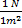Pressure is defined as the prependicular force acting per unit area.

The SI unit of pressure is Pascal (Pa) which is N/m2.
The two aspect of our daily life where pressure theory is used are: 1. Application of pressure cooker to cook our food. 2. The flow of water is highest at the tap connected at the lowest level from the tank than the subsequent taps above it.
Two factors that affect pressure are magnitude of force and surface area on wich force is applied.
It is the pressure developed in one meter square area by one newton of force.
As we know pressure is inversely proportional to the area on which the force is applied. When area is increased (keeping the force constant) the pressure is decreased as the same foce now has to act on large area so force gets dispersed.
When a man stands on one foot the surface area on which is force is acting is smaller compared to when he is standing on two foot. So, as the area is decreased the pressure applied is increased (because force is inversly proportional to the area). So, A man exerts more pressure under his foot when he stands with one foot than when he stands on two foot.
As the knife gets sharper the surface area of the knife's cutting edge have very less surface area. When the surface area is reduced the pressure applied with the same amount of force is increased as pressure is inversely proportional to the surface area. So the knife with sharp edge (having less surface area) cuts easily tahn the blunt ones (having more surface area).
The lady wearing pointed heel will exert more pressure because we know pressure is inversly proportional to the surface area and the area of the pointed is less than the flat shoe.

The hydrometer which is used to measure the density of milk is called lactometer.

Here, Area of the base (A) = 10 cm x 18 cm

= 0.1 m x 0.18 m

= 0.018$m^{2}$

Depth of water in the vessel (h) = 4 cm = 0.04 m

Density of water (d) =1000 kg/$m^{3}$

∴Force on the base (F) =?

We know that

P = dgh

= 1000 $\times$ 10$\times 0.04 = 400Nm^{-2}$

Again we that

$P =\frac {F}{A}$

∴ F =P ×A

= 400 × 0.018

=7.2N

Here, Mass of the girl (m) = 30 kg

Surface area of single shoe (A) = 100 cm2

= 1 ×10-4m2

∴ Weight(F) = mg

= 30 ×10 = 300N

We know that,

p=$\frac{F}{A}$

=$\frac{300}{1 ×10^{-4}}$

= 3 ×106pa

Hence, pressure exerted on the ground is 3 × 106pa.